General questions (2011 VCE) 1) Biogas can be generated as a by-product of many farming activities. Waste waters often contain sugars, such as glucose, which can be converted to methane. A simplified reaction sequence is given below.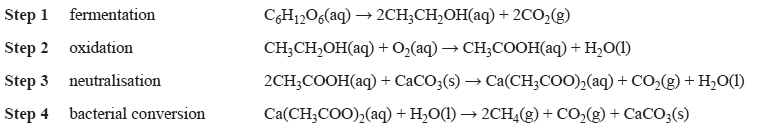The ratio of the volume of methane produced to volume of carbon dioxide produced in the overall process is a) 1 : 1 b) 1 : 2 c) 2 : 1 d) 2 : 3 Solution 2) Two titrations were performed as shown below.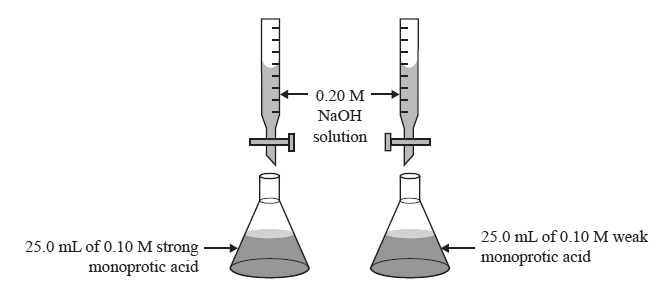Which one of the following statements is true? a) The weak acid will require a greater volume of NaOH solution than the strong acid to reach the equivalence point. b) The weak acid will require a smaller volume of NaOH solution than the strong acid to reach the equivalence point. c) The weak acid will require the same amount of NaOH solution as the strong acid to reach the equivalence point. d) The equivalence point in a ttration of a weak monoprotic acid with NaOH solution cannot be determined. Solution3) To each of three samples of a solution, a different acid-base indicator is added. The following colours are observed.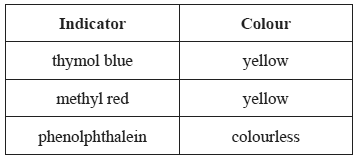The pH of the solution is between a) pH = 2.8 and pH = 4.2 b) pH = 4.2 and pH = 6.3 c) pH = 6.3 and pH = 8.3 d) pH = 8.3 and pH = 10.0 Solution 4) In an experiment, 172.1 g of gypsum, CaSO4.2H2O, (M -172.1g/mol), was heated to constant mass in a large crucible. The loss in mass of the crucible and contents was 27.0 g. The reaction that occurred when the gypsum was heated was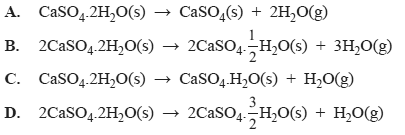Solution5) An analysis is carried out on a sample of unknown gas. The density of the gas is 2.86 grams per litre at S.T.P. The molecular formula of the gas is a) HCl b) Cl2 c) NO2 d) SO2 Solution 6) Airbags are an important safety feature of today's cars. The airbag contains a mixture of solid sodium azide, NaN3 and potassium nitrate, KNO3. In the event of an accident, trip sensors send an electric signal to an igniter. The heat generated causes the reactants to decompose completely according to the following equation.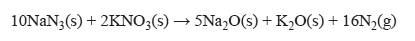A particular car's airbag was found to inflate to a volume of 62.0 L at a pressure of 100 kPa when the temperature reached 36.6 o C. The molar mass of NaN3 is 65.0 g/mol. What was the mass of sodium azide contained in the car's airbag? a) 97.9 g b) 156.6 g c) 250.6 g d) 828.1 g Solution